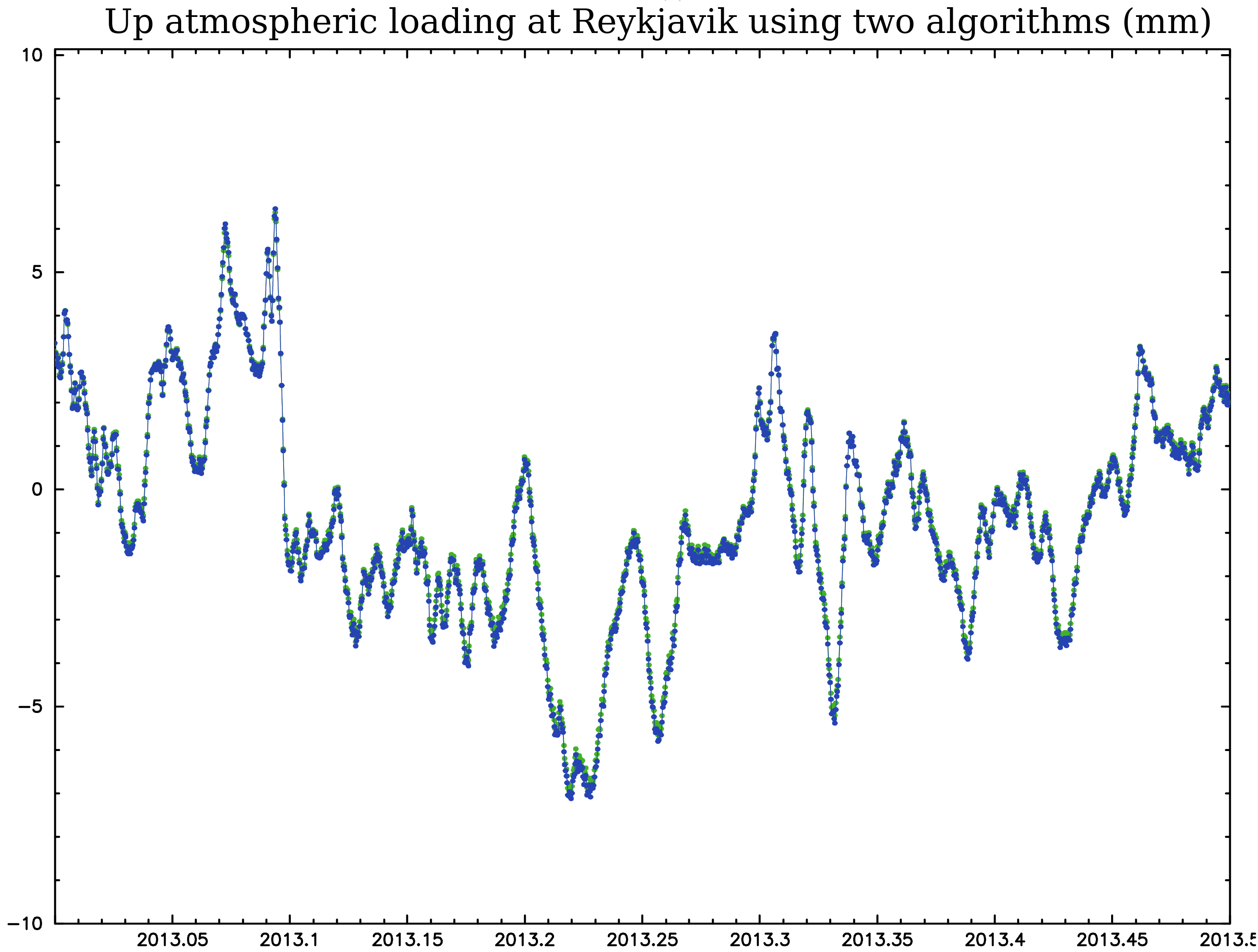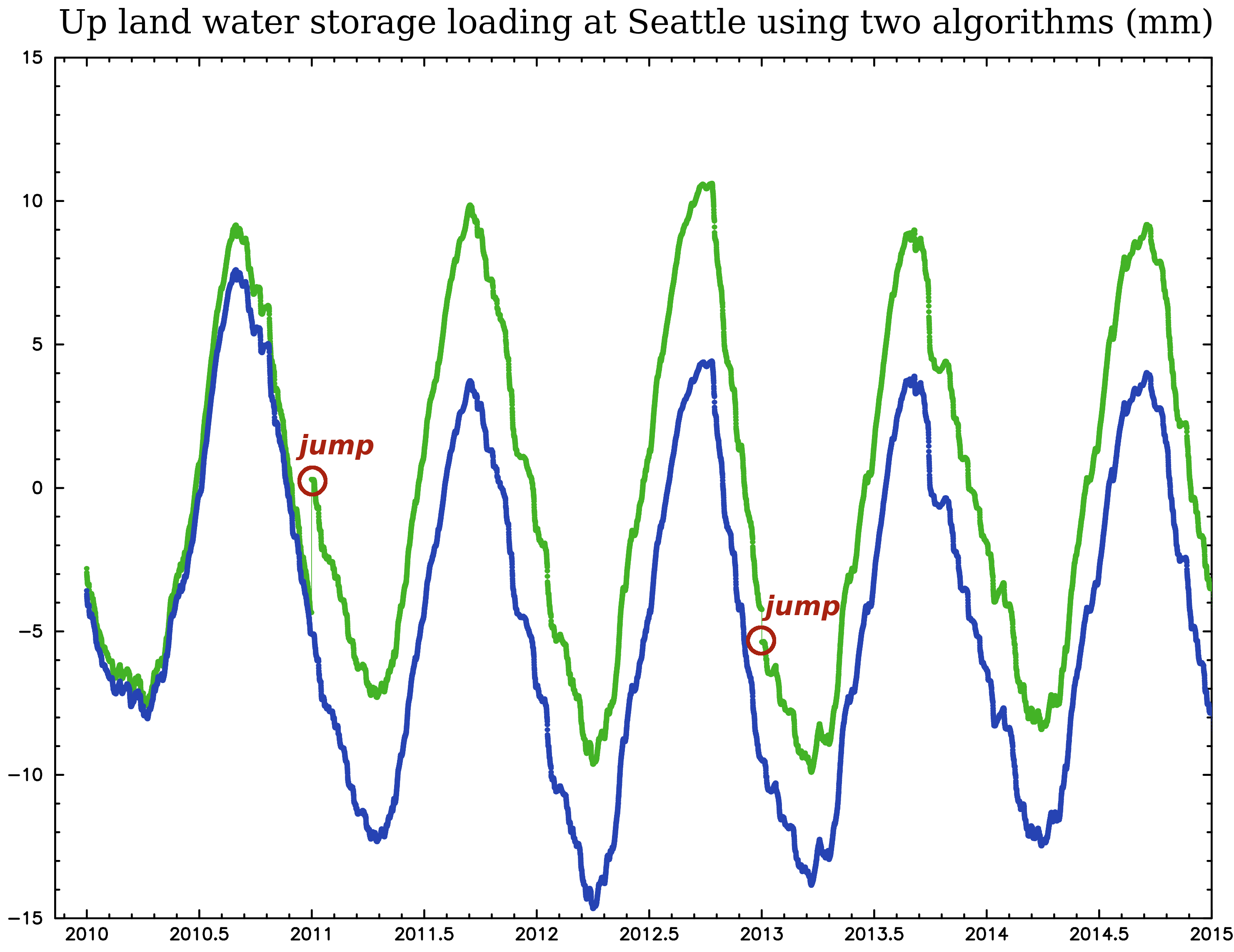##Transition of the mass loading service in June 2017

List of changes:
• The algorithm of mass loading computation was changed. The algorithm of the 3rd generation service included 1) spherical harmonic transform of the pressure fields anomaly; 2) multiplication it with h'n/(2n+1) and l'n/(2n+1) factors, where h'n and l'n are loading Love numbers; 3) storing the scaled spherical harmonics transform coefficients; 4) computation of loading displacements for a given station using the set of these coefficients. When loading at the 0.25° × 0.25° regular grid was computed, these grid elements were treated as "stations". The degree/order of the spherical; harmonics transform is 1023 (9.8 × 9.8 cos φ km grid). The spacial resolution of the 0.25° × 0.25° grid was not sufficient for interpolation within 100 km of the coastal line.

The algorithm of the 4th generation service included 1) spherical harmonic transform of the pressure fields anomaly using Driscoll-Healy sampling theorem; 2) multiplication it with h'n/(2n+1) and l'n/(2n+1) factors, 3) multiplication with the Blackmann window function; 4) inverse vector spherical harmonics transform that produce the gridded displacement field; 5) applying sampling correction that mitigate loading distortion within 1–4 cells of the coastal line; 6) storing the scaled spherical harmonics transform coefficients; 7) expansion the global displacement field into 2D B-spline basis; 7) storing the compressed coefficients of the expansion; 8) computing loading displacements at a given station with B-spline interpolation using the stored coefficients. The degree/order of the spherical; harmonics transform is 2699, i.e. the 2′ × 2′ regular longitudinal/latitudinal grid (3.7 × 3.7 cos φ km resolution).

• Main motivations of the changeover:

2. improvement of accuracy near the coastal line;
• Support of the following models was removed: MERRA-1, GEOS-FP, NOAH025, GOT-48, FES2012.
• Support of the following models was added: MERRA-2, GOT-410C, FES2014B, EQUIL01, EQUIL02. The previous version added oceanic pole tide, Sa, and model tides to GOT-48 and FES2012. The new version treats the equilibrium and dynamic zonal ocean tides separately.
• The previous version had wrong sign for FES2012 zonal ocean tides. For historical reasons the phase of zonal ocean tides has additional π term with respect to solid tide. This additional π phase is subtracted in the current version, but not corrected in the previous version.
• Jumps in land water storage surface pressure GEOS-FPIT (4 jumps) and MERRA2 (3 jumps) were estimated and eliminated from these models. As a results, the new mass loading series loading series do not have jumps.
• The limit of the number of stations for the on-demand service lifted from 256 to 16384.
• The file naming convention was changed. to 16384.
Here are example of differences of loading computation using different algorithms and same models:The differences in land water storage loading are mainly due to jumps in the soil moister models GEOS-FPIT. It affects the loading displacements computed with the previous algorithm (green points) and does not affect displacements computed with the new algorithm (blue points).

This page was prepared by Leonid Petrov ()